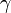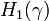# The Alexander Polynomial of a Knotted Trivalent Graph

Statement. The Alexander polynomial of a knotted trivalent graph$\gamma$ is the Reidemeister torsion of the singular homology complex of the complement of$\gamma$, with local coefficients twisted using the Alexander duality pairing with$H_1(\gamma)$. Thus is it a numerical function on$H_1(\gamma)$; in particular, if$\gamma$ is a link, it is a numerical function in as many variables as the number of components of the link. In this case it is given by a Laurant polynomial.